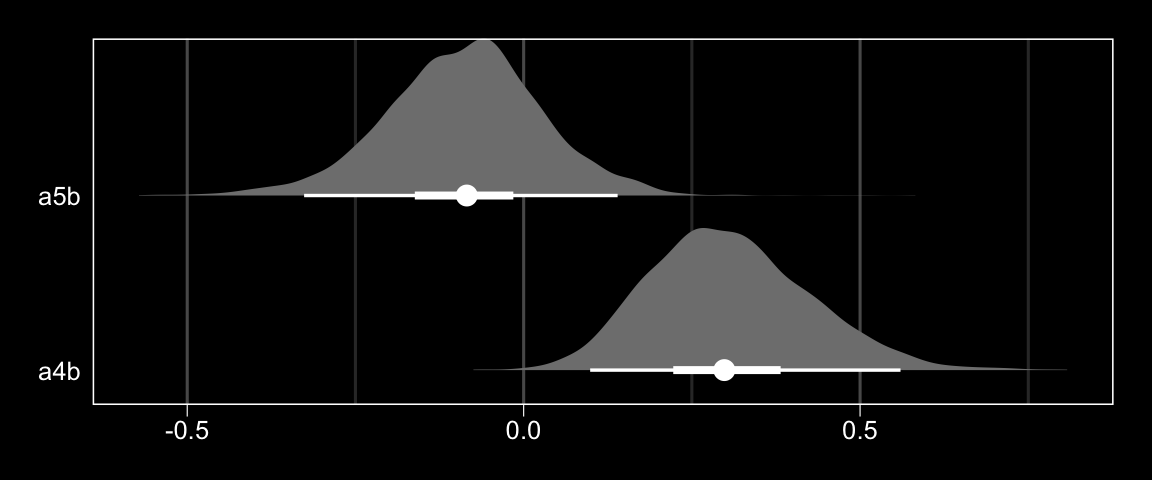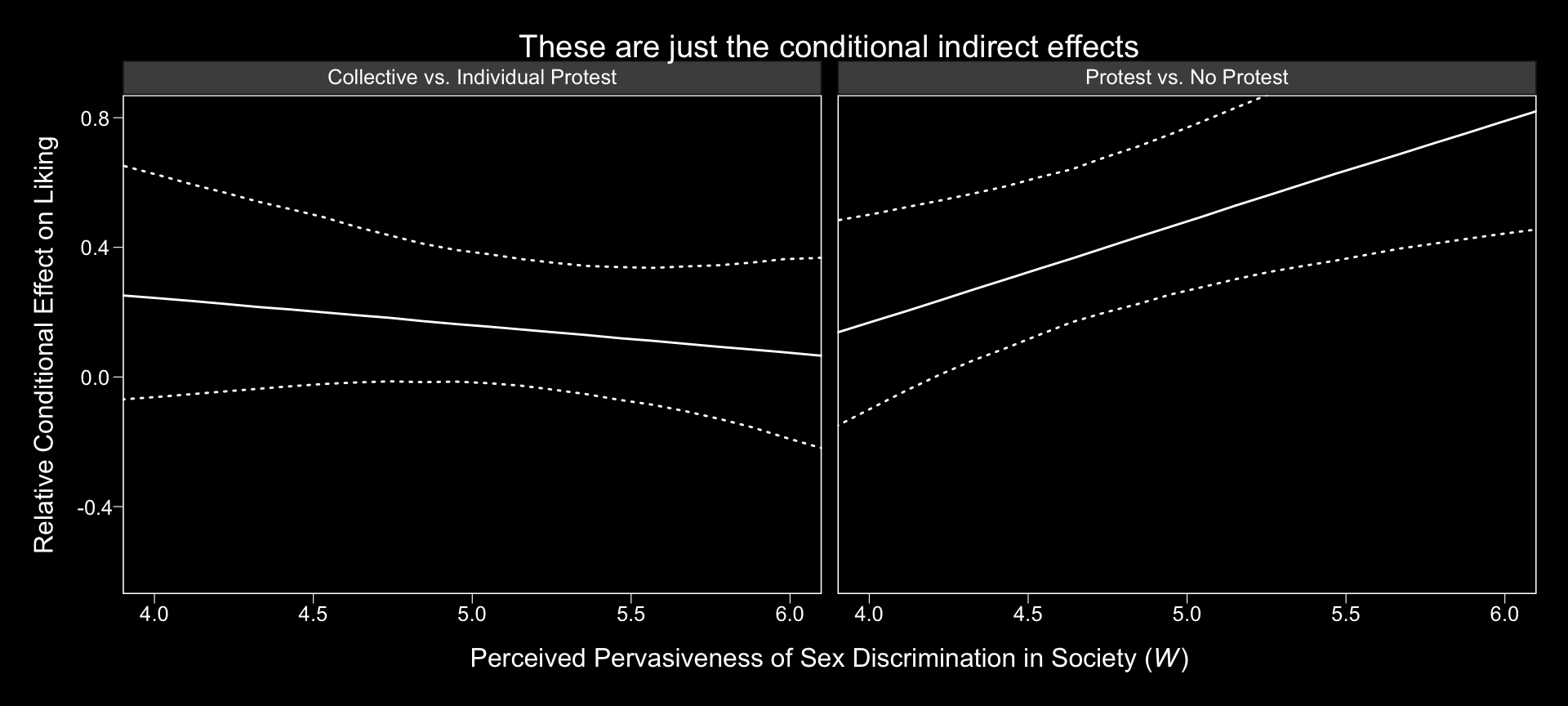## 13.4 Testing and probing moderation of mediation

### 13.4.1 A test of moderation of the relative indirect effect.

To get the slopes of Protest vs. No Protest and Collective vs. Individual Protest (i.e., $$a_{4}b$$ and $$a_{5}b$$, respectively), we just work directly with the posterior_samples(), which we’ve saved as post.

post <-
post %>%
mutate(a4b = b_respappr_D1:sexism*b_liking_respappr,
a5b = b_respappr_D2:sexism*b_liking_respappr)

post %>%
select(a4b:a5b) %>%
gather() %>%
group_by(key) %>%
summarize(mean = mean(value),
ll = quantile(value, probs = .025),
ul = quantile(value, probs = .975)) %>%
mutate_if(is.double, round, digits = 3)
## # A tibble: 2 x 4
##   key     mean     ll    ul
##   <chr>  <dbl>  <dbl> <dbl>
## 1 a4b    0.307  0.099  0.56
## 2 a5b   -0.089 -0.326  0.14

Here they are in a geom_halfeyeh() plot.

post %>%
select(a4b:a5b) %>%
gather() %>%

ggplot(aes(x = value, y = key)) +
geom_halfeyeh(point_interval = median_qi, .prob = c(0.95, 0.5),
fill = "grey50", color = "white") +
scale_y_discrete(expand = c(.1, .1)) +
labs(x = NULL, y = NULL) +
theme_black() +
theme(axis.ticks.y = element_blank(),
panel.grid.minor.y = element_blank(),
panel.grid.major.y = element_blank())### 13.4.2 Probing moderation of mediation.

We already computed the relevant 95% credible intervals at the end of section 13.3 Relative conditional indirect effects. We could inspect those in a geom_halfeyeh() plot, too.

rcie_tibble_pick_a_point %>%

ggplot(aes(x = value, y = sexism)) +
geom_halfeyeh(point_interval = median_qi, .prob = c(0.95, 0.5),
fill = "grey50", color = "white") +
labs(x = "Relative Conditional Effect on Liking", y = NULL) +
theme_black() +
theme(axis.text.y = element_text(hjust = 0),
panel.grid.minor.y = element_blank(),
panel.grid.major.y = element_blank()) +
facet_wrap(~indirect effect)